2022 AL Rookie Odds
-135
0.74 to 1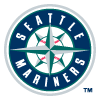Julio Rodriguez
41.7% implied probability

+360
3.6 to 1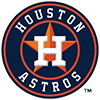Jeremy Pena
15.8% implied probability

+550
5.5 to 1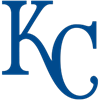Bobby Witt
11.2% implied probability

+2500
25 to 1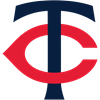Joe Ryan
2.8% implied probability

+3000
30 to 1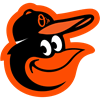Adley Rutschman
2.3% implied probability

+5000
50 to 1Riley Greene
1.4% implied probability

+5500
55 to 1Spencer Torkelson
1.3% implied probability

+5500
55 to 1Jake Burger
1.3% implied probability

+6000
60 to 1MJ Melendez
1.2% implied probability

+6500
65 to 1George Kirby
1.1% implied probability

+6500
65 to 1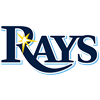Shane Baz
1.1% implied probability

+9000
90 to 1Steven Kwan
0.8% implied probability

+10000
100 to 1Richie Palacios
0.7% implied probability

+10000
100 to 1Jarren Duran
0.7% implied probability

+10000
100 to 1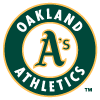A.J. Puk
0.7% implied probability

+10000
100 to 1Luis Gil
0.7% implied probability

+10000
100 to 1Xavier Edwards
0.7% implied probability

+10000
100 to 1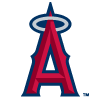Reid Detmers
0.7% implied probability

+10000
100 to 1Matt Brash
0.7% implied probability

+10000
100 to 1Kyle Isbel
0.7% implied probability

+10000
100 to 1Triston Casas
0.7% implied probability

+10000
100 to 1Jordan Groshans
0.7% implied probability

+10000
100 to 1Grayson Rodriguez
0.7% implied probability

+10000
100 to 1Tyler Nevin
0.7% implied probability

+10000
100 to 1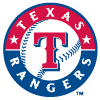Sam Huff
0.7% implied probability

+10000
100 to 1Jose Siri
0.7% implied probability

+10000
100 to 1Vidal Brujan
0.7% implied probability

+10000
100 to 1A.J. Alexy
0.7% implied probability

+10000
100 to 1Kevin Smith
0.7% implied probability

+10000
100 to 1Nick Allen
0.7% implied probability

+10000
100 to 1Nick Pratto
0.7% implied probability

+10000
100 to 1Estevan Florial
0.7% implied probability

+10000
100 to 1Gabriel Arias
0.7% implied probability

+10000
100 to 1Daulton Jefferies
0.7% implied probability

+10000
100 to 1Josh Lowe
0.7% implied probability

+10000
100 to 1Cristian Pache
0.7% implied probability

+10000
100 to 1Royce Lewis
0.7% implied probability